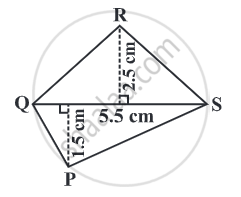# Find the area of quadrilateral PQRS. - Mathematics

Sum

Find the area of quadrilateral PQRS.#### Solution

In this case, d = 5.5 cm, h1 = 2.5cm, h2 = 1.5 cm,
Area = 1/2 d (h1 + h2)
= 1/2 xx 5.5 xx (2.5 + 1.5) cm
= 1/2 xx 5.5 xx 4 cm
= 11 cm
Concept: Area of a General Quadrilateral
Is there an error in this question or solution?